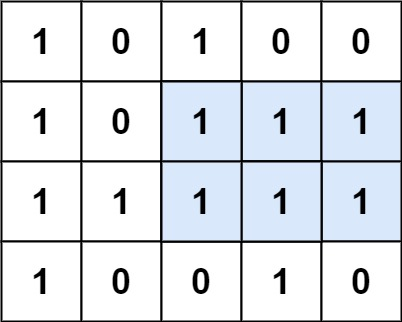## C# || Maximal Rectangle – How To Find Largest Rectangle Area Using C#The following is a module with functions which demonstrates how to find the largest rectangle area containing only 1’s using C#.

1. Maximal Rectangle – Problem Statement

Given a rows x cols binary matrix filled with 0‘s and 1‘s, find the largest rectangle containing only 1‘s and return its area.

Example 1:``` Input: matrix = [["1","0","1","0","0"],["1","0","1","1","1"],["1","1","1","1","1"],["1","0","0","1","0"]] Output: 6 Explanation: The maximal rectangle is shown in the above picture. ```

Example 2:

``` Input: matrix = [] Output: 0 ```

Example 3:

``` Input: matrix = [["0"]] Output: 0 ```

Example 4:

``` Input: matrix = [["1"]] Output: 1 ```

Example 5:

``` Input: matrix = [["0","0"]] Output: 0 ```

2. Maximal Rectangle – Solution

The following is a solution which demonstrates how to find the largest rectangle area containing only 1’s.

This solution uses the monotonic stack approach.

``` 2. Maximal Rectangle - Solution C# // ============================================================================ // Author: Kenneth Perkins // Date: Nov 30, 2021 // Taken From: http://programmingnotes.org/ // File: Solution.cs // Description: Demonstrates how to find the largest rectangle area // ============================================================================ public class Solution { public int MaximalRectangle(char[][] matrix) { if (matrix.Length == 0) { return 0; } var result = 0; var histogram = new int[matrix.Length + 1]; for (int row = 0; row < matrix.Length; ++row) { var stack = new Stack<int>(); for (int col = 0; col <= matrix[row].Length; ++col) { // Set value if (col < matrix[row].Length) { if (matrix[row][col] == '1') { histogram[col] += 1; } else { histogram[col] = 0; } } // Compute area while (stack.Count > 0 && histogram[stack.Peek()] >= histogram[col]) { var area = histogram[stack.Pop()] * (stack.Count == 0 ? col : (col - stack.Peek() - 1)); result = Math.Max(result, area); } stack.Push(col); } } return result; } }// http://programmingnotes.org/ 1234567891011121314151617181920212223242526272829303132333435363738 // ============================================================================//    Author: Kenneth Perkins//    Date:   Nov 30, 2021//    Taken From: http://programmingnotes.org///    File:  Solution.cs//    Description: Demonstrates how to find the largest rectangle area// ============================================================================public class Solution {    public int MaximalRectangle(char[][] matrix) {        if (matrix.Length == 0) {            return 0;        }         var result = 0;        var histogram = new int[matrix.Length + 1];        for (int row = 0; row < matrix.Length; ++row) {            var stack = new Stack<int>();            for (int col = 0; col <= matrix[row].Length; ++col) {                // Set value                if (col < matrix[row].Length) {                    if (matrix[row][col] == '1') {                        histogram[col] += 1;                    } else {                        histogram[col] = 0;                    }                }                 // Compute area                while (stack.Count > 0 && histogram[stack.Peek()] >= histogram[col]) {                    var area = histogram[stack.Pop()] * (stack.Count == 0 ? col : (col - stack.Peek() - 1));                    result = Math.Max(result, area);                }                stack.Push(col);            }        }        return result;    }}// http://programmingnotes.org/ ```

QUICK NOTES:
The highlighted lines are sections of interest to look out for.

The code is heavily commented, so no further insight is necessary. If you have any questions, feel free to leave a comment below.

Once compiled, you should get this as your output for the example cases:

``` 6 0 0 1 0 ```